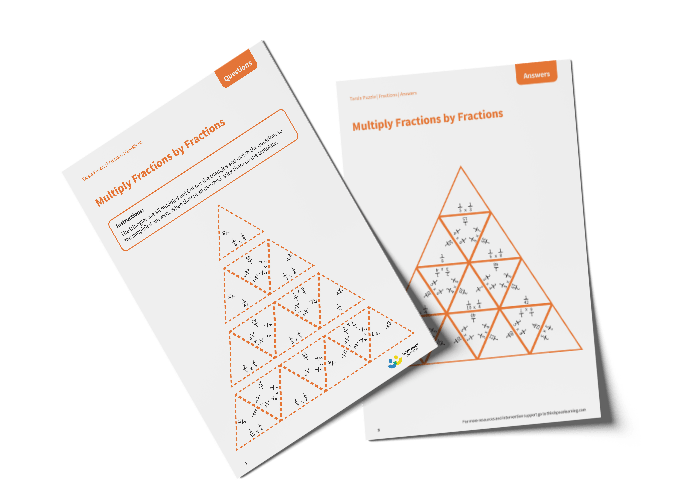# Tarsia Puzzle Multiply Fractions by Fractions (Year 6)

The triangles in the tarsia puzzle have all been mixed up. In this multiplying fractions by fractions tarsia puzzle, pupils will need to match the multiplication question to the correct answer to create one large triangle.

This Year 6 multiply fractions by fractions tarsia puzzle worksheet contains:

• One question sheet
• One template sheet

Year 6

Fractions